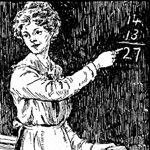# Lit2Go

## History of Modern Mathematics

### by David Eugene SmithHistory of Modern Mathematics is a comprehensive guide to different theories and strategies of mathematics ranging from Theory of Numbers, Theory of Equations, Complex Numbers, Calculus, Analytical Geometry, and others.

Source: Smith, D.E. (1906). Hisotyr of Modern Mathematics. London: Chapman and Hall.

Editor's Preface
Preface to the History of Modern Mathematics.
Author's Preface
The author’s preface to the book.
Article 1: Introduction
An introduction to the history of mathematics.
Article 2: Theory of Numbers
A discussion of the Theory of Numbers and its origin.
Article 3: Irrational and Transcendent numbers
A study of irrational and transcendent numbers and how it was first established.
Article 4: Complex Numbers
A discussion about complex numbers and an explanation of the theory.
Article 5: Quaternions and Ausdehnungslehre
A discussion and explanation of Quaternions and Ausdehnungslehre.
Article 6: Theory of Equations
A study of the Theory of Numerical Equations.
Article 7: Substitutions and Groups
A look at substitutions and groups.
Article 8: Determinants
A discussion of determinants and the theory's origin.
Article 9: Quantics
A discussion of the theory of Quantics and its origin.
Article 10: Calculus
A discussion of calculus and its origin.
Article 11: Differential Equations
A discussion of the Theory of Differential Equations and its origin and importance in mathematics.
Article 12: Infinite Series
A discussion of the Theory of Infinite Series and its development.
Article 13: Theory of Functions
A discussion of the Theory of Functions and its origin.
Article 14: Probabilities and Least Squares
A discussion of probability and least squares as well as the Theory of Probabilities and Errors.
Article 15: Analytic Geometry
A look at analytic geometry and its origin.
Article 16: Modern Geometry
A discussion of modern geometry and its evolution.
Article 17: Elementary Geometry
A look at elementary geometry.
Article 18: Non-Euclidean Geometry
A discussion of Non-Euclidean geometry and its origin and first scientific investigation.
General Tendencies
A discussion of the mathematical leaders of the nineteenth century.
• Year Published: 1906
• Language: English
• Country of Origin: United States of America